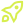Subscribe to our 0800-DEVOPS Newsletter## Not sure where to start? Let our experts guide you. Send us your query through this contact form.## Get in touch## Use the form below to apply for course## Get in touchDevelopment

# Introduction to R programming language

DURATION 2 Days
In course, participants learn about objects in R, defining and using variables and functions, basic data types, vectors and operations on vectors.

Overview

R is a specific programming language designed for data processing, statistical computing and data visualization. Besides various statistical and visualization packages it includes machine learning and advanced data processing packages.

The goal of this course is to give introduction to basic concepts of R programming language. Course is held entirely through interactive R notebooks that give course attendees unique hands on experience. In course, attendees learn about objects in R, defining and using variables and functions, basic data types, vectors and operations on vectors. R is famous for its visualization capabilities, especially using ggplot package which is learned in course. R is primarily designed as statistical language, therefore it has wide range of statistical and machine learning packages which are taught in course. Entire course lectures and exercises are done in RStudio, one of the most popular development environments for R.

Target audience

• Data Scientists
• Data analysts
• Software Engineers

Prerequisites

• Knowledge of basic programming concepts such as variables, functions and loops is desirable

Content

Day 1:

• R basics
• Objects, variables
• Functions
• Data types
• Vectors and arithmetic with vectors
• Basic data wrangling
• Programming in R basics
• Data visualization
• Introduction to data visualization
• Data distribution, quantiles, boxplots
• Plotting data with ggplot
• Summarizing data with dplyr
• Data visualization principles

Day 2:

• Data wrangling
• Tidy data – cleaning and preparing data
• String processing
• Working with dates and times
• Introduction to statistics
• Basics of statistics, probability
• Inference and modelling
• Machine learning
• Classification and regression
• Model evaluation